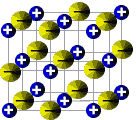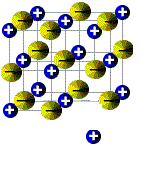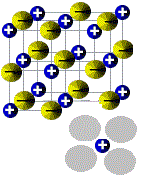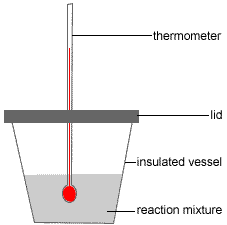Heat of Solution or Enthalpy of Solution Chemistry Tutorial

Key Concepts

⚛ A solute dissolves in excess solvent to form a solution:

solute + solvent → solution

⚛ Heat of solution, or, enthalpy of solution, is the energy released or absorbed when the solute dissolves in the solvent.

⚛ Molar heat of solution, or, molar enthalpy of solution, is the energy released or absorbed per mole of solute being dissolved in solvent.

⚛ Heat of solution (enthalpy of solution) has the symbol(1) ΔHsoln

⚛ Molar heat of solution (molar enthalpy of solution) has the units(2) J mol-1 or kJ mol-1

⚛ If heat is released when the solute dissolves, temperature of solution increases, reaction is exothermic, and ΔH is negative (ΔH < 0).

solute + solvent → solution     ΔHsoln = −

solute + solvent → solution     ΔHsoln < 0

⚛ If heat is absorbed when the solute dissolves, temperature of solution decreases, reaction is endothermic, and ΔH is positive (ΔH > 0).

solute + solvent → solution     ΔHsoln = +

solute + solvent → solution     ΔHsoln > 0

⚛ Enthalpy (heat) of solution can be determined in the laboratory by measuring the temperature change of the solvent when solute is added.

⚛ To calculate the molar enthalpy of solution (molar heat of solution) using experimental data:

Step 1: Calculate the amount of energy released or absorbed (q)

q = m × Cg × ΔT

q = amount of energy released or absorbed
m = mass
Cg = specific heat capacity
ΔT = change in temperature

Step 2: Calculate moles of solute (n)

n = m ÷ M

n = moles of solute
m = mass of solute
M = molar mass of solute

Step 3: Calculate mount of energy (heat) released or absorbed per mole of solute (ΔHsoln)

ΔHsoln = q ÷ n

ΔHsoln = molar enthalpy (heat) of solution
q = amount of energy (heat) released or absorbed
n = moles of solute

Note: You must include the sign for ΔHsoln (either + or −)

(i) ΔH is negative if energy (heat) is released (exothermic).

(ii) ΔH is positive if energy (heat) is absorbed (endothermic).

No ads = no money for us = no free stuff for you!

Theory Behind Determining Molar Enthalpy of SolutionThe molecules or ions making up a solid solute exist in a highly ordered state which is referred to as a lattice.
You are probably already familiar with representations of ionic compounds in which positive ions (cations) and negative ions (anions) are arranged in a lattice held together by electrostatic forces of attraction known as ionic bonds.
The amount of disorder, or randomness, in a system is known as its entropy. A lattice is highly ordered, that is, the particles making up the lattice are in a low state of disorder. This is referred to as a low entropy state(3).

When this solute dissolves in a solvent, particles such as ions must be removed from the lattice and each solute particle must then be completely surrounded by solvent molecules.
The solute particles in the solution are in constant motion and distributed more or less randomly throughout the solution so that the amount of disorder has increased compared to when they were part of the lattice. Solute particles in the solution are said to be in a higher state of entropy than solute particles making up the lattice.

The process of dissolving a solid solute in a liquid solvent can therefore be thought of as occurring in two steps:

 Step 1: A particle of solute, such as an ion or molecule, breaks away from the lattice. Step 2: The solute particle is surrounded by solvent molecules.Lattice energy, ΔHlat, is the energy required to break up the crystal lattice. Breaking up the lattice is an endothermic process. Solvation energy, ΔHsolv is the energy released or absorbed when solute particles are completely surrounded by solvent molecules. When water is used as the solvent, we use the term hydration energy, ΔHhyd, rather than the more general term solvation energy. Hydration is usually exothermic, so heat is released when water molecules completely surround solute particles. ΔHlat > 0 ΔHhyd < 0

If Enthalpy of Solution (heat of solution) is Endothermic:

The magnitude of the energy absorbed to break up the lattice, ΔHlat, is greater than the magnitude of the energy released when solute particles are surrounded by water solvent molecules, ΔHhyd, so the enthalpy of solution, ΔHsoln, is positive, that is, the process is endothermic.

if |ΔHlat| > |ΔHhyd| then ΔHsoln is positive

solute(s) + water(l) → solution(aq)     ΔHsoln = +

solute(s) + water(l) + ΔHsoln → solution(aq)

Energy is absorbed, that is, energy is a reactant.

When solute is added to water, water temperature decreases.

 ions ΔHlat ↑       | |       | ΔHhyd |       | |       | |       ↓ | solution |       ↑ ΔHsoln |       | salt

If Enthalpy of Solution (heat of solution) is Exothermic:

The magnitude of the energy absorbed to break up the lattice is less than magnitude of the energy released when solute particles are surrounded by water solvent molecules , so the enthalpy of solution is negative, that is, the process is exothermic.

if |ΔHlat| < |ΔHhyd| then ΔHsoln is negative

solute(s) + water(l) → solution(aq)     ΔHsoln = -

solute(s) + water(l) → solution(aq) + ΔHsoln

Energy is released, that is, energy is a product.

When solute is added to water, water temperature increases.

 ions ΔHlat ↑     | ΔHhyd |     | |     | |     | salt     | ΔHsoln |     | ↓     ↓ solution

The table below lists the values of molar enthalpy of solution (molar heat of solution) for some common salts in water at 25°C.(4)

Molar Enthalpy of Solution of Some Salts at 25°C
Endothermic examples   Exothermic examples
Anion solute ΔHosoln
(kJ mol-1)
solute ΔHosoln
(kJ mol-1)
chloride NH4Cl(s) +14.78   LiCl(s) -37.03
NaCl(s) +3.88
KCl(s) +17.22
bromide NH4Br(s) +16.78   LiBr(s) -23.26
KBr(s) +19.87 NaBr(s) -0.60
nitrate NH4NO3(s) +25.69   LiNO3(s) -2.51
AgNO3(s) +22.59
NaNO3(s) +20.50
KNO3(s) +34.89
hydroxide       LiOH(s) -23.56
NaOH(s) -44.51
KOH(s) -57.61
acetate       NH4C2H3O2(s) -2.38
NaC2H3O2(s) -17.32
KC2H3O2(s) -15.33

Experiment to Determine the Molar Heat of Solution (Molar Enthalpy of Solution) of a Solute

The following describes the use of a polystyrene foam (styrofoam™) cup as a calorimeter to determine the heat of solution of a salt (solid solute) in water (the solvent).(5)

Polystyrene foam (styrofoam™) is a good insulator, that is, it is a material that does not conduct heat well.

In this experiment the polystyrene foam (styrofoam™) cup is used as an insulated vessel in which to conduct the experiment so that either:

(i) all the energy released by the reaction is used to raise the temperature of the water in the cup and is not lost in heating the surrounding environment in the case of an exothermic reaction

(ii) the only energy being absorbed by the reaction is that from the water in the cup and not from the surrounding environment in the case of an endothermic reaction.

Procedure:1. Step 1: An accurately known quantity of water (the solvent) is placed in a well insulated vessel (eg, a polystyrene foam or styrofoam™ cup)
2. Step 2: The initial temperature of this solvent is recorded, Ti.
3. Step 3: An accurately known quantity of the solid solute is added, the vessel is sealed with a lid and the solution stirred using the thermometer.
4. Step 4: The final temperature of the solution is recorded, Tf.

Note the final temperature will be the maximum temperature reached for an exothermic reaction or the minimum temperature reached for an endothermic reaction.

Calculation of Molar Enthalpy of Solution (molar heat of solution) (6)

Step 1: Calculate the heat released or absorbed, in joules, when the solute dissolves in the solvent:

heat released or absorbed = mass × specific heat capacity × change in temperature

q = m × cg × (Tfinal - Tinitial)

q = m × cg × ΔT

 What is the value of mass, m? There are 2 ways to look at this: 1. Method usually used by High School courses. Assume all the solute dissolves simultaneously in the solvent so that all the heat is simultaneously absorbed from, or released to, the pure solvent. m = mass of solvent in grams Sometimes the volume of solvent is given rather than the mass. Convert volume to mass using the density of the liquid: density = mass ÷ volume so, mass (g) = density (g mL-1) × volume (mL) density of water at 25°C and 101.3 kPa is usually given as 1 g mL-1 So, cg = specific heat capacity of the solvent in J°C-1g-1 For water, cg = 4.18 J°C-1g-1 ΔT = Tfinal - Tinitial in °C(7) When calculated, q is in joules (J) You can convert energy in joules (J) to kilojoules (kJ) by dividing the number of joules by 1000. OR 2. Method usually used in introductory University courses. Assume the first molecule or ion of solute dissolves into pure solvent, but each subsequent molecule or ion is dissolving into a mixture of dissolved solute in solvent (that is, a solution). In this case, the final solute molecule or ion is dissolving into a solution with a mass approximated by the mass of the solvent plus the mass of the solute. m = mass of solvent + mass of solute in grams So, cg = specific heat capacity of the solution in J°C-1g-1 And, it is assumed that cg(solution) = cg(solvent) in J°C-1g-1 ΔT = Tfinal - Tinitial in °C(7) When calculated, q is in joules (J) You can convert energy in joules (J) to kilojoules (kJ) by dividing the number of joules by 1000.

Step 2: Calculate moles of solute:

where:

moles = amount of solute in mole

mass = mass of solute in grams

molar mass = molar mass of solute in grams per mole

Step 3: Calculate the molar enthalpy of solution, or molar heat of solution, ΔHsoln:

ΔHsoln in J per mole of a solute is calculated: ΔHsoln in kJ per mole of a solute is calculated:
ΔHsoln = q ÷ moles of solute (J mol-1)

where
q = energy released or absorbed as calculated in step 1.
and
moles of solute is that calculated in step 2.

exothermic reactions: ΔHsoln is negative

endothermic reactions: ΔHsoln is positive

ΔHsoln = q/1000 ÷ moles of solute (kJ mol-1)

where
q = energy released or absorbed as calculated in step 1.
and
moles of solute is that calculated in step 2.

exothermic reactions: ΔHsoln is negative

endothermic reactions: ΔHsoln is positive

Worked Example: Sample Experimental Results and Calculations

Question:
A student added 4.00 g of NaOH(s) to 100 g of water in a polystyrene foam cup.
The temperature of the water rose by 10.0°C.
Assuming the polystyrene foam cup is well insulated and the specific heat capacity of water is 4.18 J°C-1g-1, determine the molar enthalpy of solution of sodium hydroxide in kJ mol-1.

Solving the Problem using the StoPGoPS model for problem solving:

STOP STOP! State the Question.
What is the question asking you to do?

Calculate the molar heat of solution of sodium hydroxide in kJ mol-1

ΔHsoln = ? kJ mol-1

PAUSE PAUSE to Prepare a Game Plan
(1) What information (data) have you been given in the question?

m(NaOH) = mass NaOH(s) = 4.00 g

m(H2O) = mass H2O(l) = 100 g

ΔT = temperature increase = 10.0°C

cg(H2O) = specific heat capacity of water = 4.18 J°C-1g-1

(2) What is the relationship between what you know and what you need to find out?

1. heat released = q = m × cg × ΔT
2. moles of solute = n(NaOH) = m(NaOH) ÷ M(NaOH)
3. ΔHsoln = q ÷ n(NaOH)

Note: ΔHsoln will be negative because the temperature increased, that is, the process is exothermic.

GO GO with the Game Plan

 Method 1:(High School) Assume all solute dissolves simultaneously and instantaneously. Method 2:(University)Assume solute does not dissolve simultaneously and instantaneously. Step 1: Calculate heat released (kJ): heat released = q = m × cg × ΔT m = m(H2O) = 100 g cg(H2O) = 4.18 J°C-1g-1 ΔT = 10.0°C q = 100 × 4.18 × 10.0 = 4180 J q = 4180 J ÷ 1000 J/kJ = 4.18 kJ Step 2: Calculate moles of solute: moles of solute = n(NaOH) = mass(NaOH) ÷ M(NaOH) m(NaOH) = 4.00 g M(NaOH) = 22.99 + 16.00 + 1.008 = 39.998 g mol-1 n(NaOH) = 4.00 ÷ 39.998 = 0.100 mol Step 3: Calculate &Delt;Hsoln ΔHsoln = q ÷ n(NaOH) ΔHsoln is negative (process is exothermic) q = 4.18 kJ n(NaOH) = 0.100 mol ΔHsoln = -4.18 ÷ 0.100 = -41.8 kJ mol-1 (Note: only 3 significant figures are justified) Step 1: Calculate heat released (kJ): heat released = q = m × cg × ΔT m = m(H2O) + m(NaOH) = 100 + 4.00 = 104 g assume cg(solution) = cg(H2O) = 4.18 J°C-1g-1 ΔT = 10.0°C q = 104 × 4.18 × 10.0 = 4347 J q = 4347 J ÷ 1000 J/kJ = 4.35 kJ Step 2: Calculate moles of solute moles of solute = n(NaOH) = mass(NaOH) ÷ M(NaOH) m(NaOH) = 4.00 g M(NaOH) = 22.99 + 16.00 + 1.008 = 39.998 g mol-1 n(NaOH) = 4.00 ÷ 39.998 =0.100 mol Step 3: Calculate ΔHsoln ΔHsoln = q ÷ n(NaOH) ΔHsoln is negative (process is exothermic) q = 4.35 kJ n(NaOH) = 0.100 mol ΔHsoln = -4.35 ÷ 0.100 = -43.5 kJ mol-1 (Note: only 3 significant figures are justified)
PAUSE PAUSE to Ponder Plausibility

Work backwards:
Assuming ΔHsoln ≈ -42 kJ mol-1 = 42,000 J mol-1, what temperature change is expected if 4 g of NaOH is dissolved in 100 g water?

 ΔHsoln = q ÷ n(NaOH) rearrange: q = ΔHsoln × n(NaOH) substitute m(NaOH)/M(NaOH) for n(NaOH) q = ΔHsoln × m(NaOH)/M(NaOH) substitute m × cg × ΔT for q m × cg × ΔT = ΔHsoln × m(NaOH)/M(NaOH) rearrange: ΔT = [ΔHsoln × m(NaOH)/M(NaOH)]/[m × cg] substitute values: ΔT = = = ≈ [42000 × 4.00/(23 + 16 + 1)]/[100 × 4.18] [42000 × 0.1]/ 4200/418 10°C

Since this value for the change in temperature is the same as that given in the question, we are confident our answer is correct.

STOP STOP! State the Solution
State your solution to the problem.

Method 1: Molar enthalpy of solution of sodium hydroxide is −41.8 kJ mol-1
ΔHsoln(NaOH) = −41.8 kJ mol-1

Method 2: Molar enthalpy of solution of sodium hydroxide is −43.5 kJ mol-1
ΔHsoln(NaOH) = −43.5 kJ mol-1

Sample Question

A student added 1.92 g of KBr(s) to 100 g of water in an insulated vessel. The temperature of the water fell by 0.766°C. Calculate ΔHsoln in kJ mol-1.

ΔHsoln = kJ mol-1

Footnotes:

(1) If the solute and the solvent are in their standard states, you can also write ΔHosol
Refer to Standard Enthalpy (heat) of Formation and Reaction

(2) You may also units of cal mol-1 or kcal mol-1
1 calorie = 4.18 joules
1 cal = 4.18 J
For conversions between J, kJ, and cal refer to energy conversions

(3) Entropy refers to the amount of disorder, or randomness, in a system.
Ions locked into a crystal structure are in a low state of disorder and therefore have low entropy.
Ions moving randomly through the solvent molecules in a solution are much more disordered, much more random, so they are said to be in a higher state of entropy.
Gas molecules moving randomly in the atmosphere are in an even greater state of disorder or randomness, so they are said to have an even higher state of entropy.
Moving towards a higher state of entropy is one of the driving forces for a chemical reaction.

(4) The values given are for solutions at infinite dilution which is the enthalpy change when 1 mole of solute in its standard state is dissolved in an infinite amount of water.

(5) Go to Calorimetry Tutorial to see an alternative method used an adiabatic solution calorimeter.

(6) On this page the calculations assume that there is a large excess of solvent
Note that the following examples all use the specific heat capacity of the solvent, cg, not molar heat capacities, C.

(7) You can use other units for temperature.
If you use Kelvin (K), then cg will have the same numbers but the units change to JK-1g-1. This because a change of temperature of 1K is the same as the change of temperature of 1°C.
If you use fahrenheit then you will have to use a value for cg that has the units J°F-1g-1